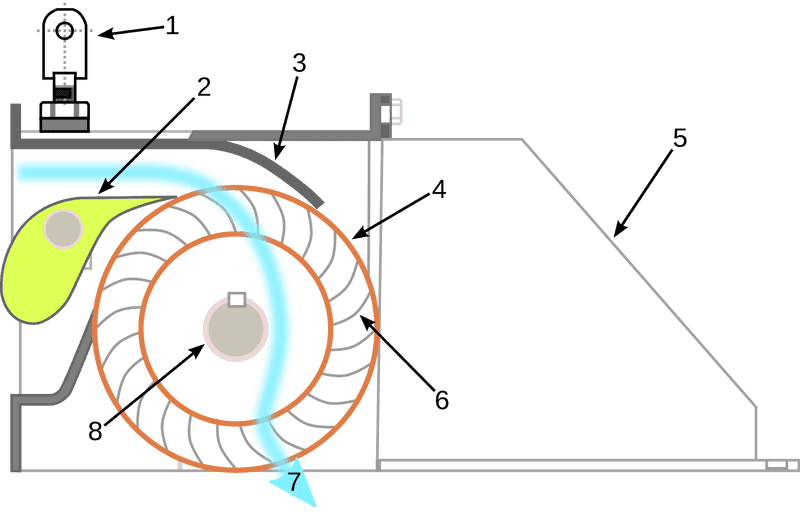# How to Calculate the Speed of a Sprocket?

How to calculate the speed of sprocket connected to the turbine shaft of a cross flow turbine if I only have these given parameters: Net Head, Volume Flow Rate, Specific Weight of Water and Efficiency of Cross Flow Turbine? Does the Cross Flow Turbine, Turbine Shaft and Speed of Sprocket have the same speed? Then, what formulas should I use to finally compute the speed of Sprocket?

How to calculate the speed of sprocket connected to the turbine shaft of a cross flow turbine if I only have these given parameters: Net Head, Volume Flow Rate, Specific Weight of Water and Efficiency of Cross Flow Turbine? Does the Cross Flow Turbine, Turbine Shaft and Speed of Sprocket have the same speed? Then, what formulas should I use to finally compute the speed of Sprocket?
I think, if the efficiency is given, power out of the turbine can be calculated by using the water power.Then the rpm of the shaft can be calculated from the output power.
If the sprocket is attached to the shaft with a key, then it should rotate at the same speed as the shaft.This is for no load condition.

Last edited:
anorlunda
Staff Emeritus
How is the sprocket connected? Via a belt? a gear?

In this thread, and your previous thread, you did not mention the load on the turbine. What is the turbine driving? The more mechanical load you put on the turbine, the slower the RPM for the same power.

•russ_watters
jack action
Gold Member
The rpm always depends on the torque output.

What you know is the power output. In your case it is ##P = \eta\rho g h \dot{V}##, where ##\eta## is the efficiency of your turbine, ##\rho## is the fluid density, ##g## is the acceleration due to gravity and ##\dot{V}## is the volume flow rate. This will always be constant.

As @anorlunda mentioned, you then need to know the load you will put on your turbine, i.e. what is the torque output ##T##? Then ##rpm = \frac{P}{T}##. If you put no load on the turbine, then it will accelerate until it reaches a sufficient load ... or until it self-destructs.

russ_watters
Mentor
How to calculate the speed of sprocket connected to the turbine shaft of a cross flow turbine if I only have these given parameters: Net Head, Volume Flow Rate, Specific Weight of Water and Efficiency of Cross Flow Turbine? Does the Cross Flow Turbine, Turbine Shaft and Speed of Sprocket have the same speed? Then, what formulas should I use to finally compute the speed of Sprocket?
It can't be calculated with the information given. The rotation speed of a turbine is dependent on the specifics of the design of the turbine and does not have an easy analytical solution unless you already know every other directly related parameter about the turbine's performance (and you know almost nothing about the turbine; mostly you just know about the water).

This question has the feel of being a part of a larger issue becuase it isn't a very meaningful/useful question in and of itself. Is this a real world problem? Please tell us what the real problem is.

If you put no load on the turbine, then it will accelerate until it reaches a sufficient load ... or until it self-destructs.

Does this mean, the friction resistance will be the only load.Since it is comparitively small, the turbine will attain very high speeds and after a limit it self destructs due to the high temperature attained(due to friction)?Does the friction resistance increases with speed?

jack action
Gold Member
Does this mean, the friction resistance will be the only load.
Yes.

Since it is comparitively small, the turbine will attain very high speeds and after a limit it self destructs due to the high temperature attained(due to friction)?
Yes. But sometimes, the turbine could be so off from its design characteristics, that the power output will be significantly lowered before self-destruction is reached.

Does the friction resistance increases with speed?
Yes. Just considering a simple bearing, you can see that speed is a factor.

•Mohankpvk and russ_watters
CWatters
Homework Helper
Gold Member
You said you know the volume flow rate through the turbine. Do you also know the volume per revolution of the turbine?

anorlunda
Staff EmeritusThe speed won't increase forever, no matter how little friction you have. There will be a speed at which no more power is extracted from the water in this arrangement because the vertical velocity of falling water will match the vertical component of the blade at the given blade angle. You can't make that exact balance simultaneously on both the top and bottom blades because the water velocity is different top and bottom. However, you can see that energy extracted from the water (thus turbine efficiency) will vary greatly with wheel speed with efficiency approaching zero at "high" speed.

So, to add to what @russ_watters told you, there's not enough information to do the calculation. One of the missing things is the shape and angle of the blades.

If this is a school project, I suggest that you do experiments rather than calculations.

#### Attachments

•Mohankpvk and russ_watters